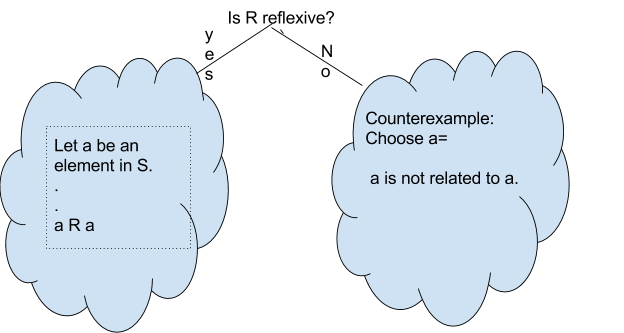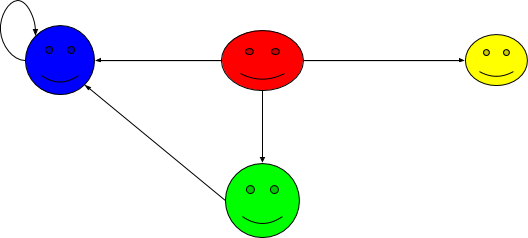# 0.2: Relations

$$\newcommand{\vecs}{\overset { \rightharpoonup} {\mathbf{#1}} }$$ $$\newcommand{\vecd}{\overset{-\!-\!\rightharpoonup}{\vphantom{a}\smash {#1}}}$$$$\newcommand{\id}{\mathrm{id}}$$ $$\newcommand{\Span}{\mathrm{span}}$$ $$\newcommand{\kernel}{\mathrm{null}\,}$$ $$\newcommand{\range}{\mathrm{range}\,}$$ $$\newcommand{\RealPart}{\mathrm{Re}}$$ $$\newcommand{\ImaginaryPart}{\mathrm{Im}}$$ $$\newcommand{\Argument}{\mathrm{Arg}}$$ $$\newcommand{\norm}{\| #1 \|}$$ $$\newcommand{\inner}{\langle #1, #2 \rangle}$$ $$\newcommand{\Span}{\mathrm{span}}$$ $$\newcommand{\id}{\mathrm{id}}$$ $$\newcommand{\Span}{\mathrm{span}}$$ $$\newcommand{\kernel}{\mathrm{null}\,}$$ $$\newcommand{\range}{\mathrm{range}\,}$$ $$\newcommand{\RealPart}{\mathrm{Re}}$$ $$\newcommand{\ImaginaryPart}{\mathrm{Im}}$$ $$\newcommand{\Argument}{\mathrm{Arg}}$$ $$\newcommand{\norm}{\| #1 \|}$$ $$\newcommand{\inner}{\langle #1, #2 \rangle}$$ $$\newcommand{\Span}{\mathrm{span}}$$$$\newcommand{\AA}{\unicode[.8,0]{x212B}}$$

Definition: Binary Relation

Let $$S$$ be a nonempty set. Then any subset $$R$$ of $$S \times S$$ is said to be a relation over $$S$$. In other words, a relation is a rule that is defined between two elements in $$S$$. Intuitively, if $$R$$ is a relation over $$S$$, then the statement $$a R b$$ is either true or false for all $$a,b\in S$$.

If the statement $$a R b$$ is false, we denote this by $$a \not R b$$.

Example $$\PageIndex{1}$$:

Let $$S=\{1,2,3\}$$. Define $$R$$ by $$a R b$$ if and only if $$a < b$$, for $$a, b \in S$$.

Then $$1 R 2, 1 R 3, 2 R 3$$ and $$2 \not R 1$$.

We can visualize the above binary relation as a graph, where the vertices are the elements of S, and there is an edge from $$a$$ to $$b$$ if and only if $$a R b$$, for $$a b\in S$$.The following are some examples of relations defined on $$\mathbb{Z}$$.

Example $$\PageIndex{2}$$:

1. Define $$R$$ by $$a R b$$ if and only if $$a < b$$, for $$a, b \in \mathbb{Z}$$.
2. Define $$R$$ by $$a R b$$ if and only if $$a >b$$, for $$a, b \in \mathbb{Z}$$.
3. Define $$R$$ by $$a R b$$ if and only if $$a \leq b$$, for $$a, b \in \mathbb{Z}$$.
4. Define $$R$$ by $$a R b$$ if and only if $$a \geq b$$, for $$a, b \in \mathbb{Z}$$.
5. Define $$R$$ by $$a R b$$ if and only if $$a = b$$, for $$a, b \in \mathbb{Z}$$.

Definition: Divisor/Divides

Let $$a$$ and $$b$$ be integers. We say that $$a$$ divides $$b$$ is denoted $$a\mid b$$, provided we have an integer $$m$$ such that $$b=am$$. In this case we can also say the following:

• $$b$$ is divisible by $$a$$
• $$a$$ is a factor of $$b$$
• $$a$$ is a divisor of $$b$$
• $$b$$ is a multiple of $$a$$

If $$a$$  does not divide $$b$$, then it is  is denoted by $$a \not \mid b$$.

## Properties of binary relation:

Definition: Reflexive

Let $$S$$ be a set and $$R$$ be a binary relation on $$S$$. Then $$R$$ is said to be reflexive if $$a R a, \forall a \in S.$$Example $$\PageIndex{7}$$:

Define $$R$$ by $$a R b$$ if and only if $$a < b$$, for $$a, b \in \mathbb{Z}$$. Is $$R$$ reflexive?

Counter Example:

Choose $$a=2.$$

Since $$2 \not< 2$$, $$R$$ is not reflexive.

Example $$\PageIndex{8}$$:

Define $$R$$ by $$a R b$$ if and only if $$a \mid b$$, for $$a, b \in \mathbb{Z}$$. Is $$R$$ reflexive?

Proof:

Let $$a \in \mathbb{Z}$$. Since $$a=(1) (a)$$, $$a \mid a$$.

Thus $$R$$ is reflexive. $$\Box$$

Definition: Symmetric

Let $$S$$ be a set and $$R$$ be a binary relation on $$S$$. Then $$R$$ is said to be symmetric if the following statement is true:

$$\forall a,b \in S$$, if $$a R b$$ then $$b R a$$, in other words, $$\forall a,b \in S, a R b \implies b R a.$$

Example $$\PageIndex{8}$$: Visually$$\forall a,b \in S, a R b \implies b R a.$$ holds!Example $$\PageIndex{9}$$:

Define $$R$$ by $$a R b$$ if and only if $$a < b$$, for $$a, b \in \mathbb{Z}$$. Is $$R$$ symmetric?

Counter Example:

$$1<2$$ but $$2 \not < 1$$.

Example $$\PageIndex{10}$$:

Define $$R$$ by $$a R b$$ if and only if $$a \mid b$$, for $$a, b \in \mathbb{Z}$$. Is $$R$$ symmetric?

Counter Example:

$$2 \mid 4$$ but $$4 \not \mid 2$$.

Definition: Transitive

Let $$S$$ be a set and $$R$$ be a binary relation on $$S$$. Then $$R$$ is said to be transitive if the following statement is true

$$\forall a,b,c \in S,$$ if $$a R b$$ and $$b R c$$, then $$a R c$$.

In other words, $$\forall a,b,c \in S$$, $$a R b \wedge b R c \implies a R c$$.

Example $$\PageIndex{14}$$: VISUALLY$$\forall a,b,c \in S$$, $$a R b \wedge b R c \implies a R c$$ holds!Example $$\PageIndex{15}$$:

Define $$R$$ by $$a R b$$ if and only if $$a < b$$, for $$a, b \in \mathbb{Z}$$. Is $$R$$ transitive?

Example $$\PageIndex{16}$$:

Define $$R$$ by $$a R b$$ if and only if $$a \mid b$$, for $$a, b \in \mathbb{Z_+}$$. Is $$R$$ transitive?

Definition: Equivalence Relation

A binary relation is an equivalence relation on a nonempty set $$S$$ if and only if the relation is reflexive(R), symmetric(S) and transitive(T).

Definition: Equivalence Relation

Definition: Equivalence Class

Given an equivalence relation $$R$$ over a set $$S,$$ for any $$a \in S$$ the equivalence class of a is the set $$[a]_R =\{ b \in S \mid a R b \}$$, that is
$$[a]_R$$ is the set of all elements of S that are related to $$a$$.

Example $$\PageIndex{3}$$: Equivalence relation

Define a relation that two shapes are related iff they are the same color. Is this relation an equivalence relation?

Equivalence classes are:Example $$\PageIndex{4}$$:

Define a relation that two shapes are related iff they are similar. Is this relation an equivalence relation?

Equivalence classes are:##### Definition: Partition

Let $$A$$ be a nonempty set. A partition of $$A$$ is a set of nonempty pairwise disjoint sets whose union is A.

Note

For every equivalence relation over a nonempty set $$S$$, $$S$$ has a partition.

For the following examples, determine whether or not each of the following binary relationson the given setis reflexive, symmetric, antisymmetric, or transitive. If a relation has a certain property, prove this is so; otherwise, provide a counterexample to show that it does not. Ifis an equivalence relation, describe the equivalence classes of.

Recall:

Let $$m,n \in \mathbb{Z}$$.

We say that $$m$$ divides $$n$$ (written as $$m|n$$) if $$n=mk$$ for some $$k\in \mathbb{Z}$$.

##### Example $$\PageIndex{1}$$

Let $$S= \mathbb{Z}, m\in \mathbb{Z}_+$$.  Define $$aRb$$ if and only if $$m|(a-b), \forall a,b \in \mathbb{Z}$$.

Note that $$m$$ is a positive integer.

Let $$m=3$$.  Choose $$a=1$$ and $$b=2$$.

Are 1 and 2 related? i.e. does $$3|1-2$$?  Does $$1-2=3k, k\in \mathbb{Z}$$?  Since $$\notexists k$$ s.t. $$-1 = 3k$$, ∴ $$1 !R 2$$.

However, we can see that we have three groups as shown in the image below:Definition: Modulo

Let $$m$$ $$\in$$ $$\mathbb{Z_+}$$.

$$a$$ is congruent to $$b$$ modulo $$m$$ denoted as $$a \equiv b (mod \, n)$$, if $$a$$ and $$b$$  have the remainder when they are divided by $$n$$, for  $$a, b \in \mathbb{Z}$$.

## Properties

Let $$n \in \mathbb{Z_+}$$. Then

#### Theorem 1 :

Two integers $$a$$ and $$b$$ are said to be congruent modulo $$n$$, $$a \equiv b (mod \, n)$$, if all of the following are true:

a) $$m\mid (a-b).$$

b) both $$a$$ and $$b$$ have the same remainder when divided by $$n.$$

c) $$a-b= kn$$, for some $$k \in \mathbb{Z}$$.

NOTE: Possible remainders of $$n$$ are $$0, ..., n-1.$$

### Reflexive Property

#### Theorem 2:

The relation " $$\equiv$$ " over $$\mathbb{Z}$$ is reflexive.

Proof: Let $$a \in \mathbb{Z}$$.

Then $$a-a=0(n)$$, and $$0 \in \mathbb{Z}$$.

Hence $$a \equiv a (mod \, n)$$.

Thus congruence modulo n is Reflexive.

### Symmetric Property

#### Theorem 3:

The relation " $$\equiv$$ " over $$\mathbb{Z}$$ is symmetric.

Proof: Let $$a, b \in \mathbb{Z}$$ such that $$a \equiv b$$ (mod n).

Then $$a-b=kn,$$ for some $$k \in \mathbb{Z}$$.

Now $$b-a= (-k)n$$ and $$-k \in \mathbb{Z}$$.

Hence $$b \equiv a(mod \, n)$$.

Thus the relation is symmetric.

### Antisymmetric Property

Is the relation " $$\equiv$$ " over $$\mathbb{Z}$$ antisymmetric?

Counter Example: $$n$$ is fixed

choose: $$a= n+1, b= 2n+1$$, then

$$a \equiv b(mod \, n)$$ and $$b \equiv a (mod \, n)$$

but $$a \ne b.$$

Thus the relation " $$\equiv$$ "on $$\mathbb{Z}$$ is not antisymmetric.

### Transitive Property

#### Theorem 4 :

The relation " $$\equiv$$ " over $$\mathbb{Z}$$ is transitive.

Proof: Let $$a, b, c \in$$ $$\mathbb{Z}$$, such that $$a \equiv b (mod n)$$ and $$b \equiv c (mod n).$$

Then $$a=b+kn, k \in$$ $$\mathbb{Z}$$ and $$b=c+hn, h \in$$ $$\mathbb{Z}$$.

We shall show that $$a \equiv c (mod \, n)$$.

Consider $$a=b+kn=(c+hn)+kn=c+(hn+kn)=c+(h+k)n, h+k \in$$ $$\mathbb{Z}$$.

Hence $$a \equiv c (mod \, n)$$.

Thus congruence modulo $$n$$ is transitive.

#### Theorem 5:

The relation " $$\equiv$$ " over $$\mathbb{Z}$$ is an equivalence relation.

## Modulo Arithmetic

In this section, we will explore arithmetic operations in a modulo world.

Let $$n \in \mathbb{Z_+}$$.

### Theorem 5 :

Let $$a, b, c,d, \in \mathbb{Z}$$ such that $$a \equiv b (mod\,n)$$ and $$c \equiv d (mod\, n).$$ Then $$(a+c) \equiv (b+d)(mod\, n).$$

Proof:

Let $$a, b, c, d \in\mathbb{Z}$$, such that $$a \equiv b (mod\, n)$$ and $$c \equiv d (mod \,n).$$

We shall show that $$(a+c) \equiv (b+d) (mod\, n).$$

Since $$a \equiv b (mod\, n)$$ and $$c \equiv d (mod\, n), n \mid (a-b)$$ and $$n \mid (c-d)$$

Thus $$a= b+nk,$$ and $$c= d+nl,$$ for $$k$$ and $$l \in \mathbb{Z}$$.

Consider$$(a+c) -( b+d)= a-b+c-d=n(k+l), k+l \in \mathbb{Z}$$.

Hence $$(a+c)\equiv (b+d) (mod \,n).\Box$$

### Theorem 6:Let $$a, b, c,d, \in \mathbb{Z}$$ such that $$a \equiv b (mod \, n)$$ and $$c \equiv d (mod \,n).$$ Then $$(ac) \equiv (bd) (mod \,n).$$

Proof:

Let $$a, b, c, d \in \mathbb{Z}$$, such that $$a \equiv b (mod\, n)$$ and $$c \equiv d (mod \, n).$$

We shall show that $$(ac) \equiv (bd) (mod\, n).$$

Since $$a \equiv b (mod \,n)$$ and $$c \equiv d (mod\, n), n \mid (a-b)$$ and $$n \mid (c-d)$$

Thus $$a= b+nk,$$ and $$c= d+nl,$$ for $$k$$ and $$l \in \mathbb{Z}$$.

Consider $$(ac) -( bd)= ( b+nk) ( d+nl)-bd= bnl+dnk+n^2lk=n (bl+dk+nlk),$$ where $$(bl+dk+nlk) \in \mathbb{Z}$$.

Hence $$(ac) \equiv (bd) (mod \,n).\Box$$

### Theorem 7:

Let $$a, b \in$$ $$\mathbb{Z}$$ such that $$a \equiv b (mod \, n)$$. Then $$a^2 \equiv b^2 (mod \, n).$$

Proof:

Let $$a, b \in$$ $$\mathbb{Z}$$, and n $$\in$$ $$\mathbb{Z_+}$$, such that $$a \equiv b (mod \, n).$$

We shall show that $$a^2 \equiv b^2 (mod \, n).$$

Since $$a \equiv b (mod \, n), n\mid (a-b).$$

Thus $$(a-b)= nx,$$ where $$x \in$$ $$\mathbb{Z}$$.

Consider $$(a^2 - b^2) = (a+b)(a-b)=(a+b)(nx), = n(ax+bx), ax+bx \in \mathbb{Z}$$.

Hence $$n \mid a^2 - b^2,$$ therefore $$a^2 \equiv b^2 (mod \, n).$$ $$\Box$$

### Theorem 8:

Let $$a, b \in$$ $$\mathbb{Z}$$ such that $$a \equiv b (mod \, n)$$. Then $$a^m \equiv b^m (mod \, n)$$, $$\forall \in$$ $$\mathbb{Z}$$.

Proof:

Exercise.

By using the above result we can solve many problems. Some of them are discussed below:

Example $$\PageIndex{1}$$: $$mod\, 3$$ Arithmetic

Let $$n = 3$$.

 +               

Multiplication

 x               

Example $$\PageIndex{2}$$: $$mod\, 4$$ Arithmetic

Let $$n=4$$.

 x                        

Example $$\PageIndex{3}$$:

Find the remainder when $$(101)(103)(107)(109)$$ is divided by $$11.$$

$$101 \equiv 2 (mod \, 11)$$

$$103 \equiv 4 (mod \, 11)$$

$$107 \equiv 8 (mod \, 11)$$

$$109 \equiv 10 (mod \,11)$$.

Therefore,

$$(101)(103)(107)(109) \equiv (2)(4)(8)(10) (mod \,11) \equiv 2 (mod \,11)$$.

Example $$\PageIndex{4}$$:

Find the remainder when$$7^{1453}$$ is divided by $$8.$$

$$7^0 \equiv 1 (mod \, 8)$$

$$7^1 \equiv 7 (mod \, 8)$$

$$7^2 \equiv 1 (mod \, 8)$$

$$7^3 \equiv 7 (mod \, 8)$$,

As there is a consistent pattern emerging and we know that $$1453$$ is odd, then $$7^{1453} \equiv 7 (mod \, 8)$$. Thus the remainder is $$7.$$

Example $$\PageIndex{5}$$:

Find the remainder when $$7^{2020}$$ is divided by $$18.$$

$$7^0 \equiv 1 (mod \, 18)$$

$$7^1 \equiv 7 (mod \, 18)$$

$$7^2 \equiv 13 (mod \, 18)$$

$$7^3 \equiv 1 (mod \, 18)$$,

As there is a consistent pattern emerging and we know that $$2020=(673)3+1$$, $$7^{2020}= 7^{(673)3+1}=\left( 7^3\right)^{673}7^1 \equiv 7 (mod \, 18).$$ Thus the remainder is $$7.$$

Example $$\PageIndex{6}$$:

Find the remainder when $$26^{1453}$$ is divided by $$3.$$

This page titled 0.2: Relations is shared under a CC BY-NC-SA 4.0 license and was authored, remixed, and/or curated by Pamini Thangarajah.